Maths-
General
Easy

Question

# Use the equations represented in the graph below to find the point of intersection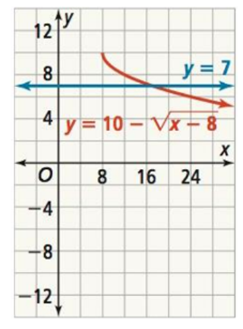Hint:

## The correct answer is: (x, y) = (17,7)

### Complete step by step solution:Here the given equations arey = 7…(i)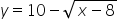…(ii)On equating (i) and (ii),we get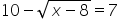On rearranging, we get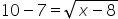On squaring both the sides, we get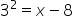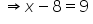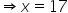So the point of intersection is (x , y) = (17,7)#### With Turito Foundation.#### Get an Expert Advice From Turito.